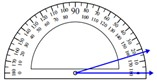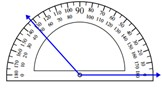# Measuring an angle with the protractor

An angle is formed when two lines intersect in a point. The rays emerging from the common point called vertex are the arms of the angle.The magnitude of how big or small an angle is given by its measure in degrees or some other units

• We place the midpoint of the protractor on the vertex of the angle.

• Then we line up one side of the angle with the zero line of the protractor.

• We then read the degrees where the other side crosses the number scale.

Measure the following angle using a protractor.### Solution

Step 1:

Line up one ray with 0°. Read the angle measurement where the other ray crosses the protractor.

Step 2:

Reading from the protractor, the given angle measures 17°.

Measure the following angle using a protractor.### Solution

Step 1:

Line up one ray with 0°. Read the angle measurement where the other ray crosses the protractor.

Step 2:

Reading from the protractor, the given angle measures 132°.